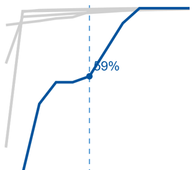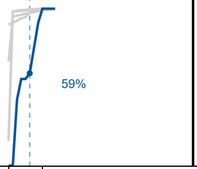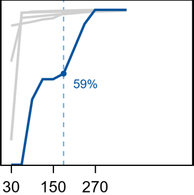## Placement of data labels PROC TEMPLATE using DATALABELPOSITION seems incorrect

Hello,

I am attempting to edit the placement of data labels in a SERIES graph in PROC TEMPLATE. The default settings get me to 95% of what I need, but when I specify the DATALABELPOSITION option, results are not as I would expect and the plot becomes unusable.

Below is one panel that is problematic using the default settings (note the collision with the line):Here is the same panel after specifying DATALABELPOSITION=BOTTOMRIGHT, thinking the label would simply move down slightly:No matter what I try for DATALABELPOSITION, anything other than AUTO scrunches up the graph. Am I missing something that's making it put the label outside the graph/plot region? Pared down code is below.

``````proc template;
define statgraph NAME;
dynamic VAR;
begingraph / designWidth=2700px designHeight=675px;
layout dataLattice columnVar=COLVAR /
rowAxisOpts=(offsetmin=0 linearopts=(tickvalueformat=percent11.0 viewmin=0) display=(tickvalues ticks label))
columnAxisOpts=(display=(tickvalues ticks label));
layout prototype;
referenceline x=XREF / lineattrs=(pattern=2 thickness=1);
< More seriesplot statements >
seriesplot x=XVAR y=YVAR / yaxis=y lineattrs=(color=&PLOTCOLOR thickness=2);
seriesplot x=XVAR y=YVAR / yaxis=y display=(markers) markerattrs=(color=&PLOTCOLOR symbol=circleFilled)
datalabel=YVAR2 datalabelattrs=(color=&PLOTCOLOR) datalabelposition=bottomright;
endlayout;
endlayout;
endgraph;
end;
run;``````

5 REPLIES 5DanH_sas
SAS Super FREQ

## Re: Placement of data labels PROC TEMPLATE using DATALABELPOSITION seems incorrect

Can you include the removed SERIESPLOT statements from your template? Also, what is the format length of your YVAR2 variable?

## Re: Placement of data labels PROC TEMPLATE using DATALABELPOSITION seems incorrect

Thanks for the reply. The omitted SERIESPLOT statements are variants of the following:

seriesplot x=XVAR y=CATEGORY1 / yaxis=y lineattrs=(color=CXD0D0D0 thickness=2);
...
seriesplot x=XVAR y=CATEGORY5 / yaxis=y lineattrs=(color=CXD0D0D0 thickness=2);

YVAR2 is a percent11.0 format with length of 8 (same as YVAR and CATEGORY1 -- CATEGORY5).
XVAR is also a number (best12) with length of 8.DanH_sas
SAS Super FREQ

## Re: Placement of data labels PROC TEMPLATE using DATALABELPOSITION seems incorrect

What do you get if you change the percentage format field width from 11 to 5?

## Re: Placement of data labels PROC TEMPLATE using DATALABELPOSITION seems incorrect

Great, thanks for the suggestion, I appreciate the assistance. That definitely made a difference, but there is still white space for about a third of the plot. Would you have an idea what else I might be missing?DanH_sas
SAS Super FREQ

## Re: Placement of data labels PROC TEMPLATE using DATALABELPOSITION seems incorrect

Also, set OFFSETMAX=0 (or 0.05) on your rowAxisOpts.

Discussion stats
• 5 replies
• 715 views
• 0 likes
• 2 in conversation